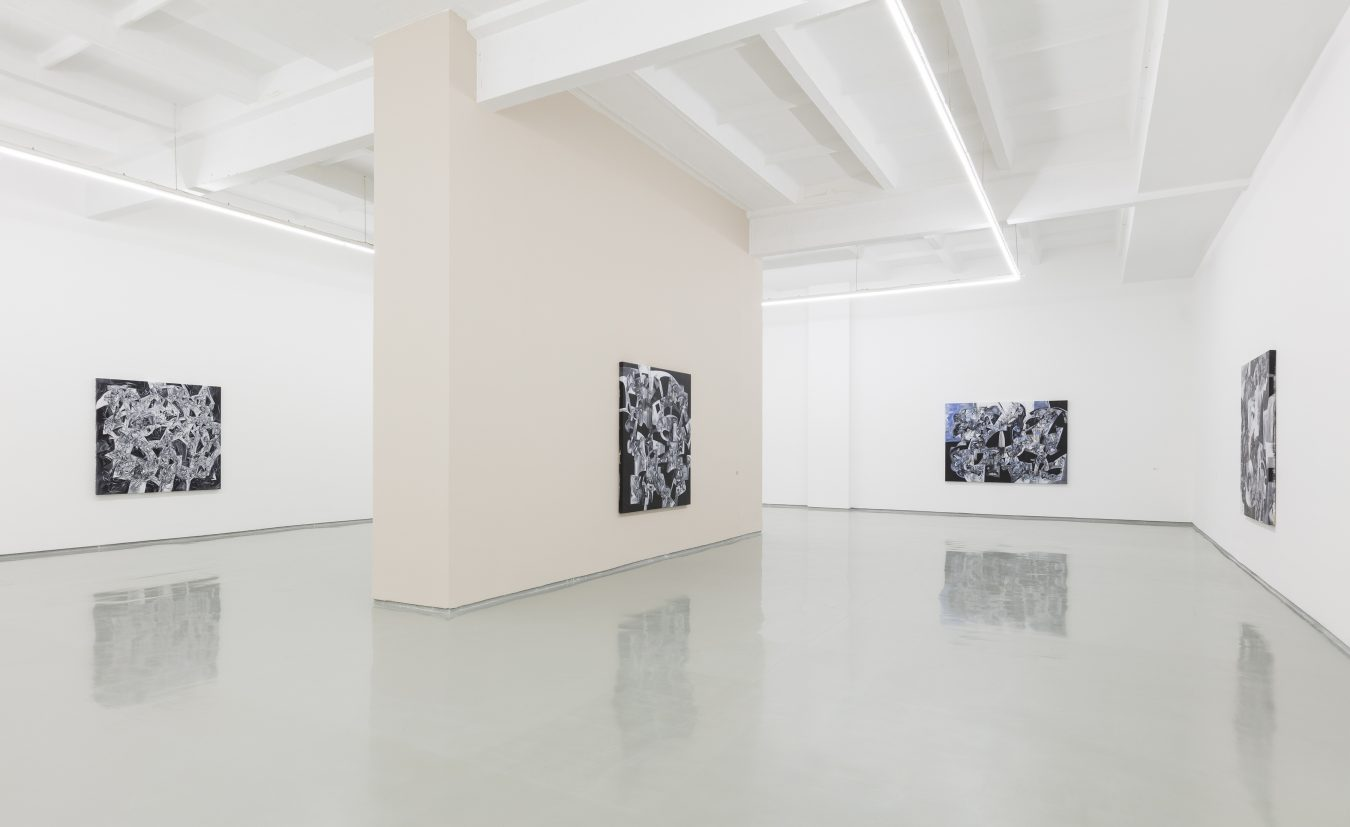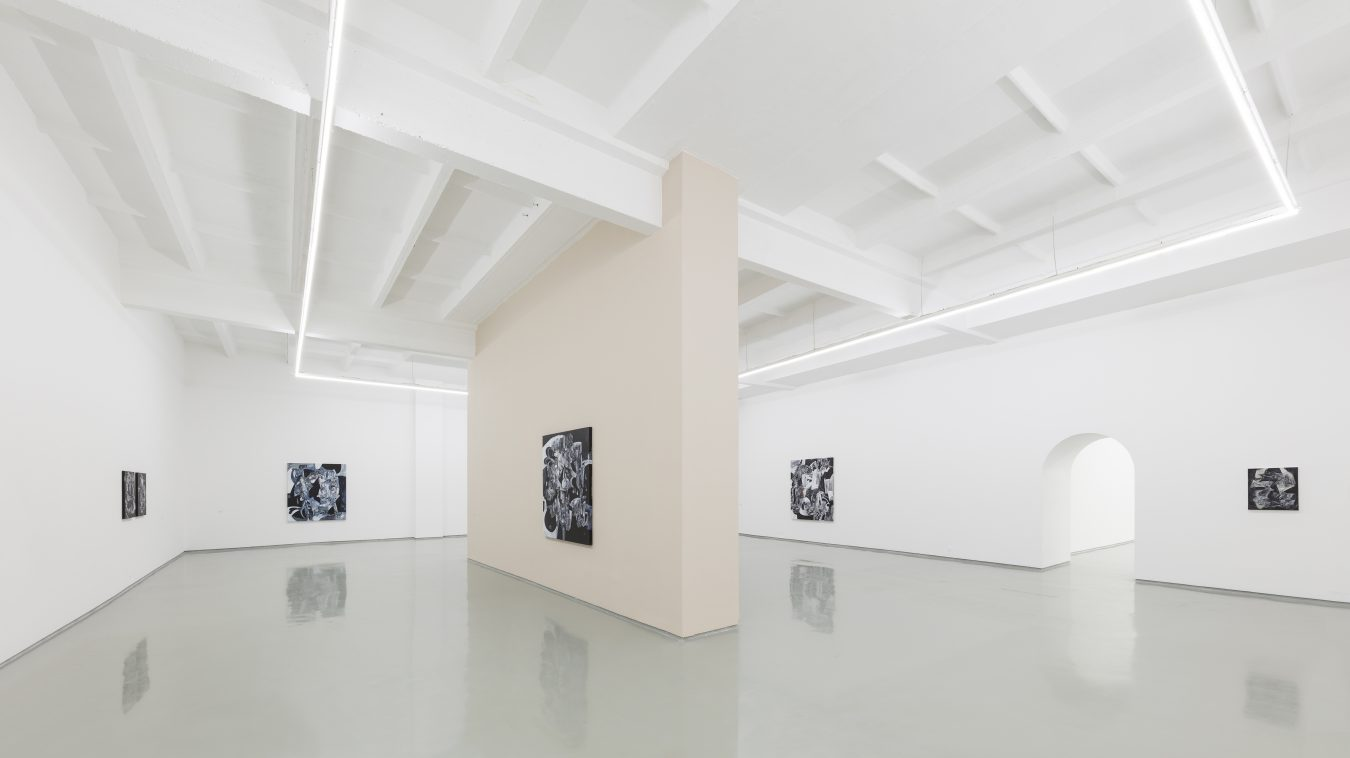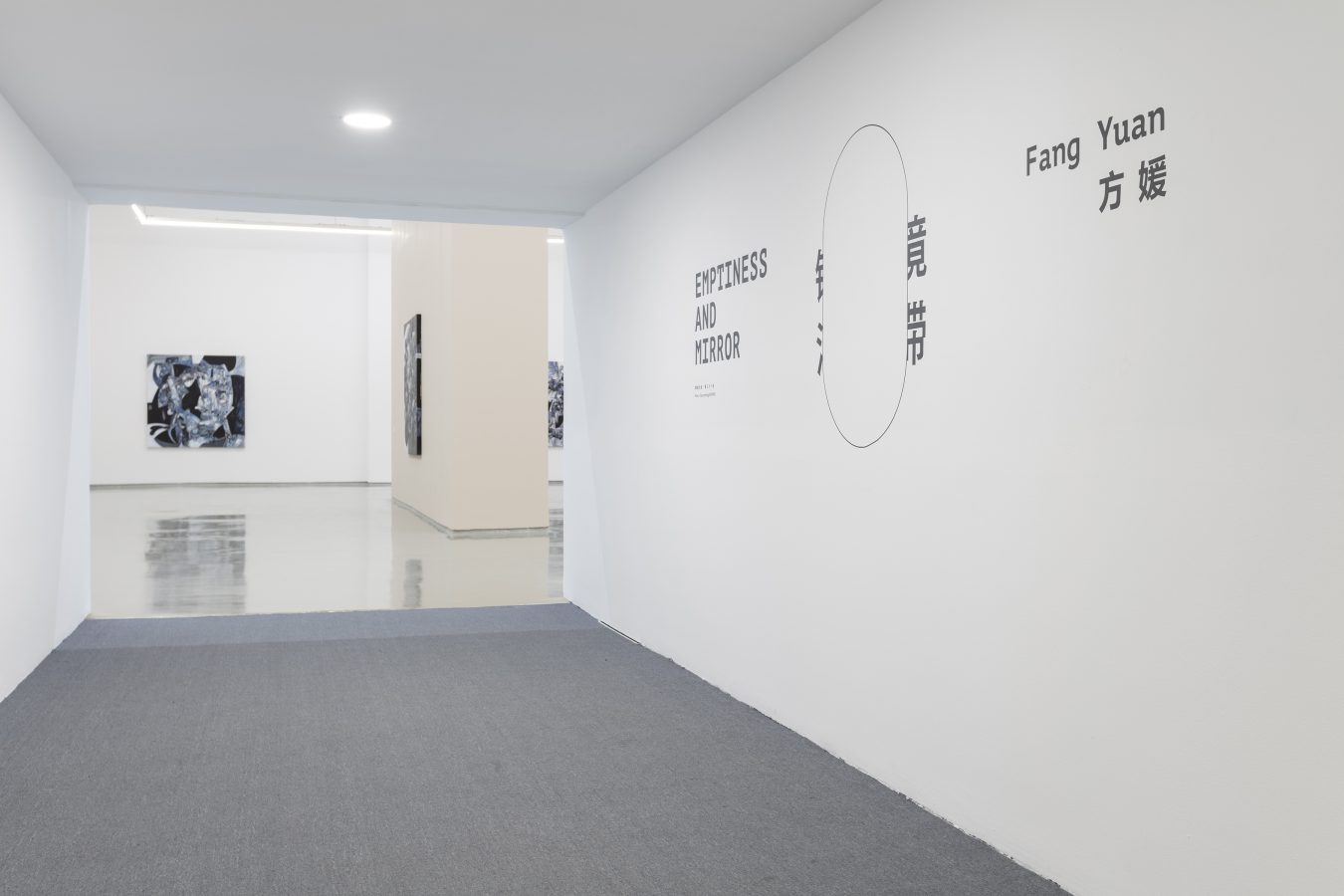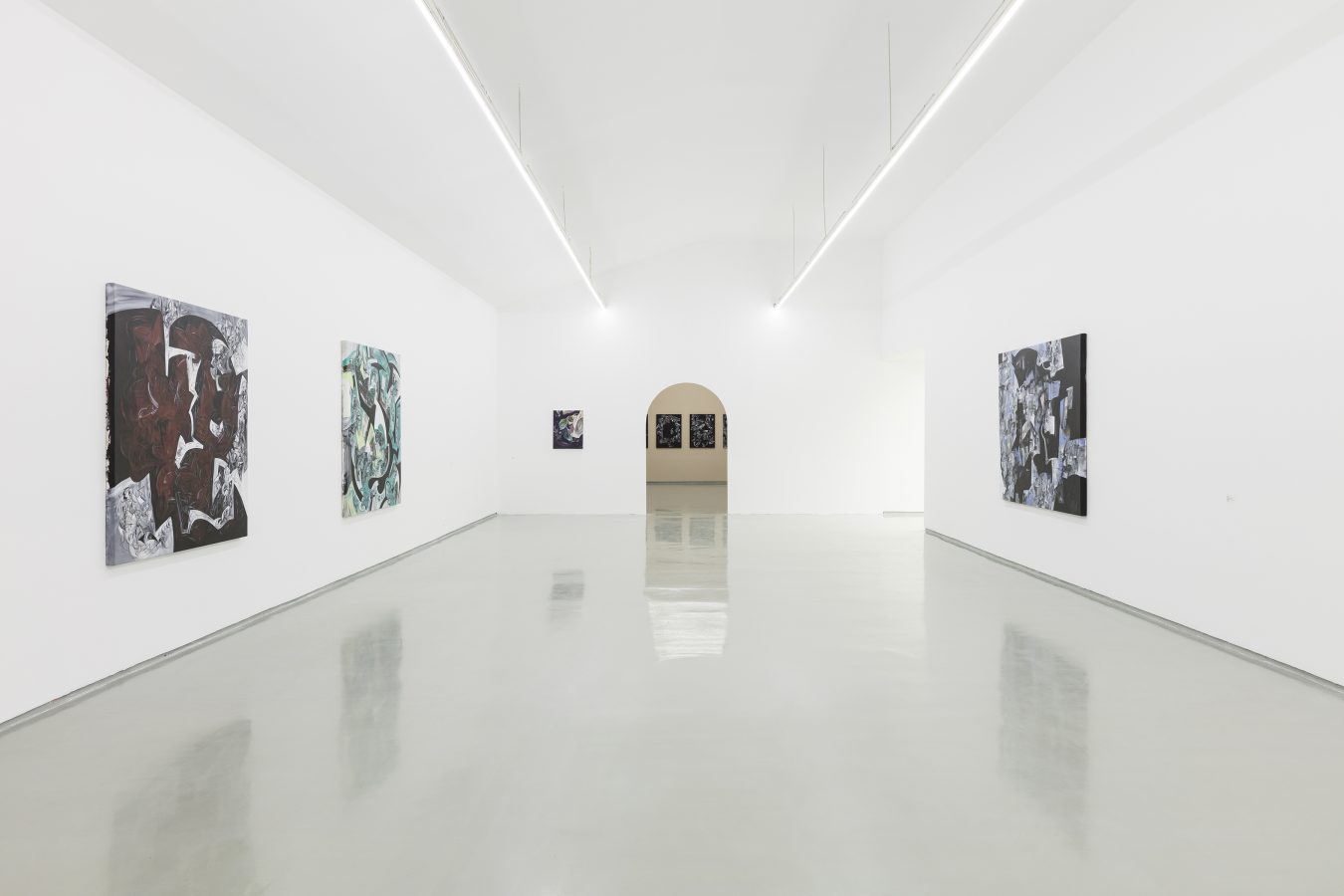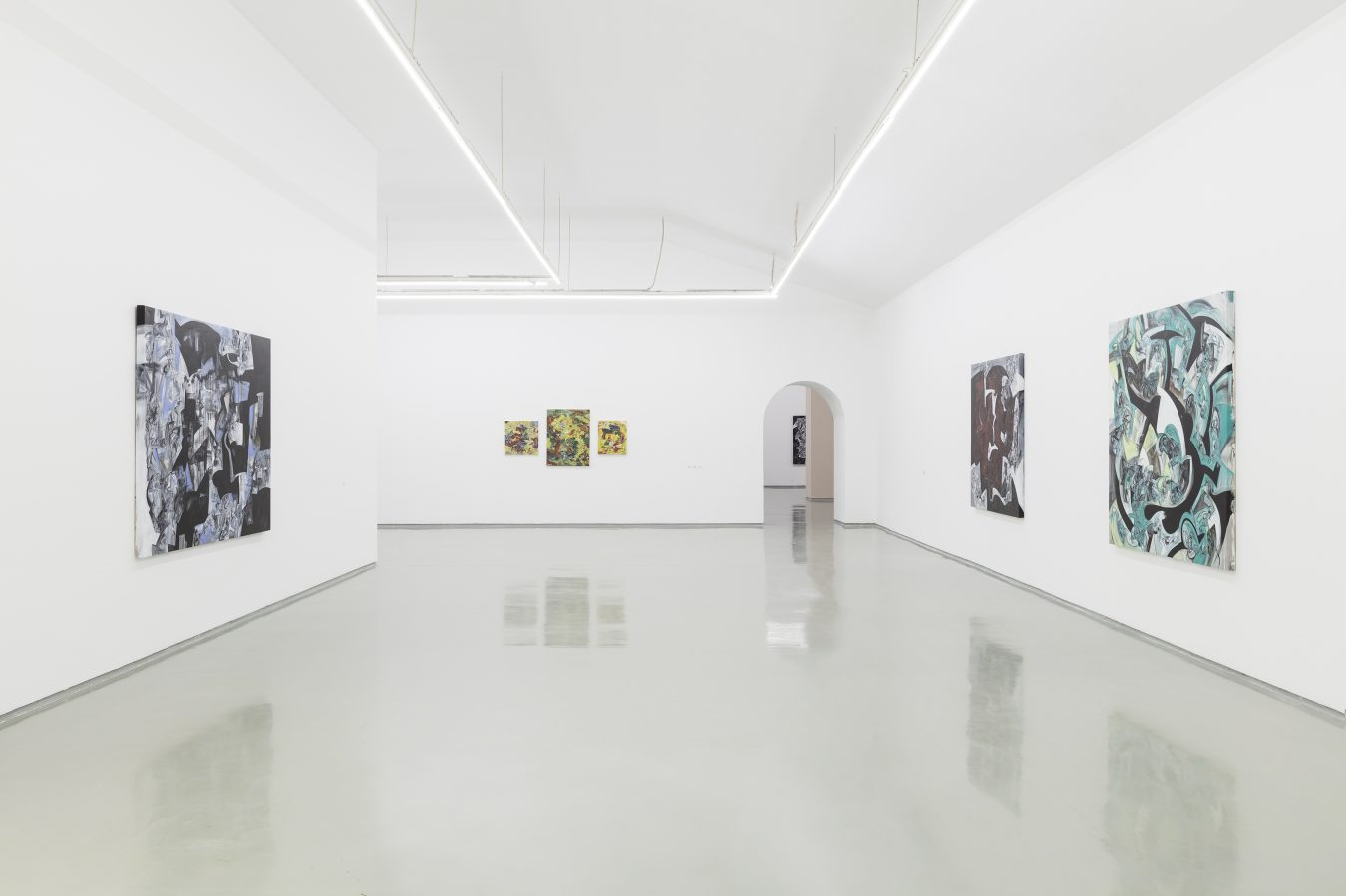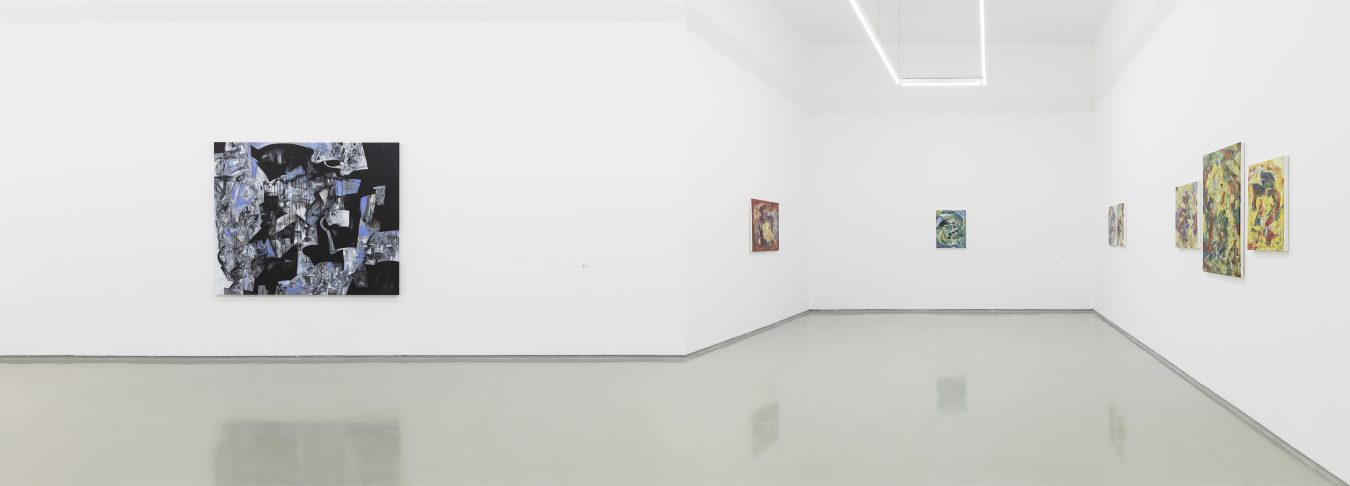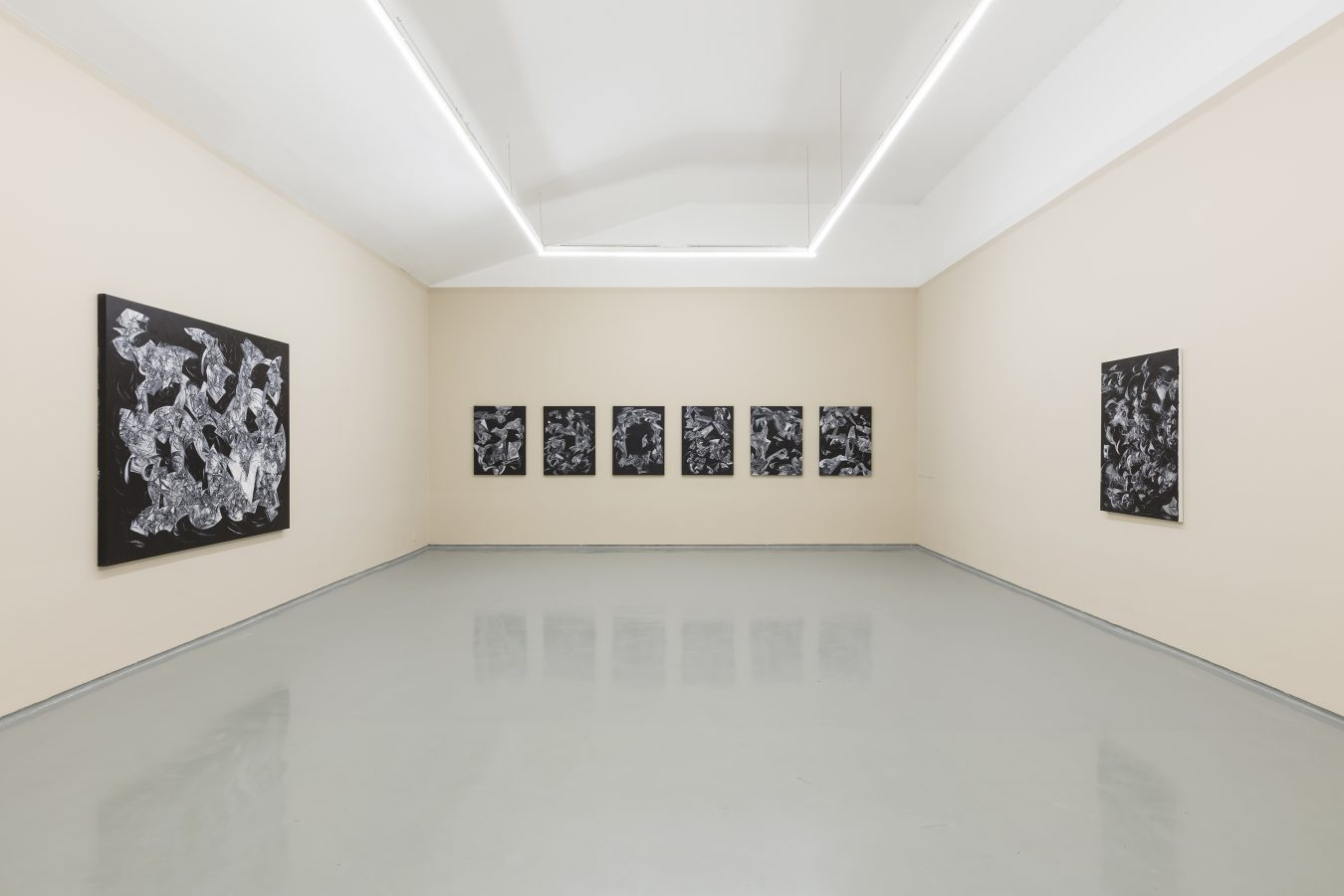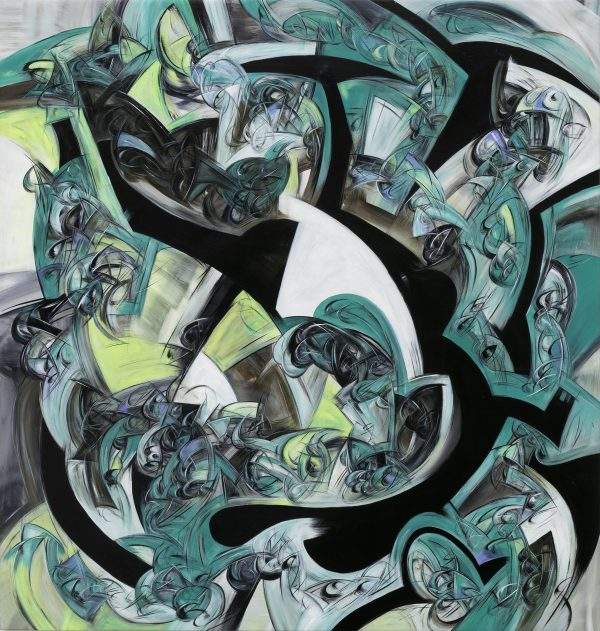2021. 布面丙烯. 176×166cm.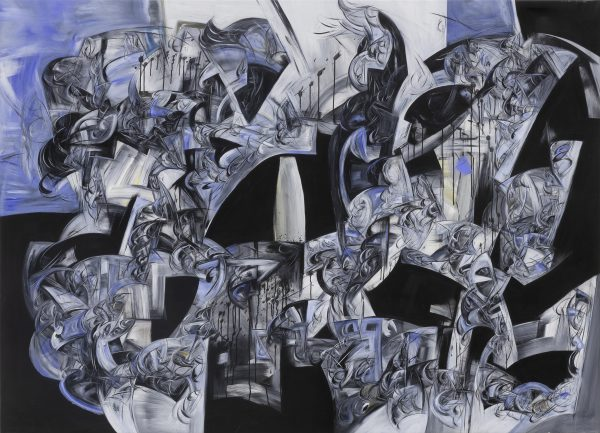2021. 布面丙烯与印度墨. 166×230cm.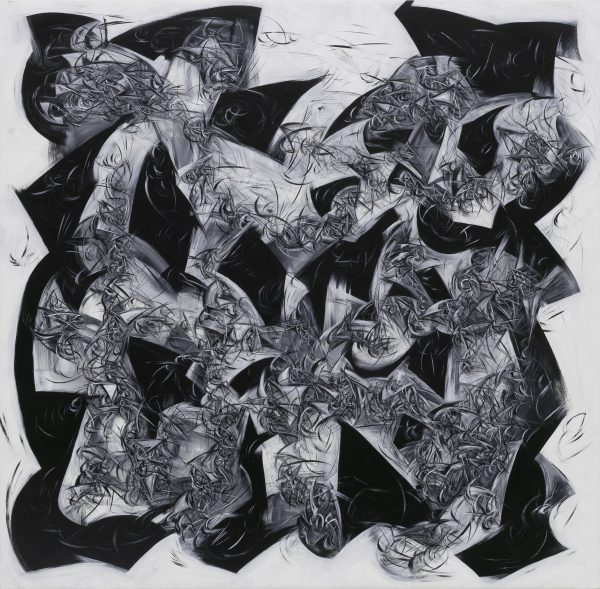2021. 布面丙烯. 162×165cm.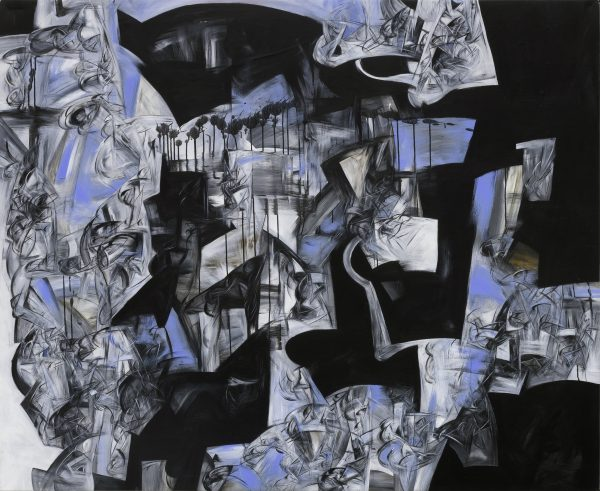2021. 布面丙烯与印度墨. 166×203cm.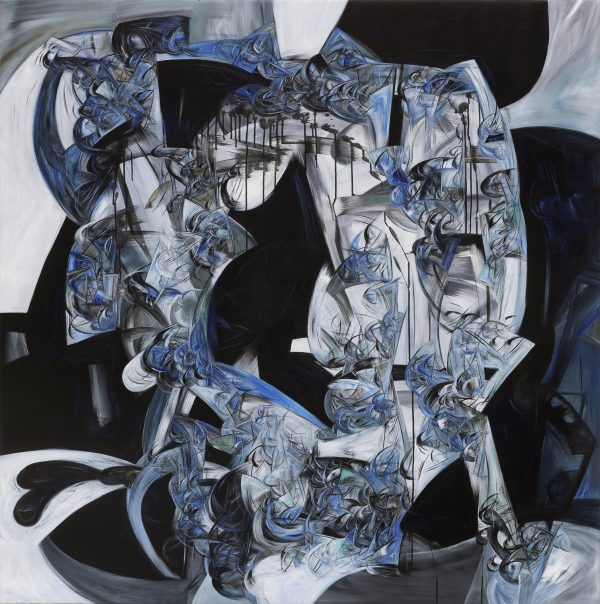2021. 布面丙烯与印度墨. 167×166cm.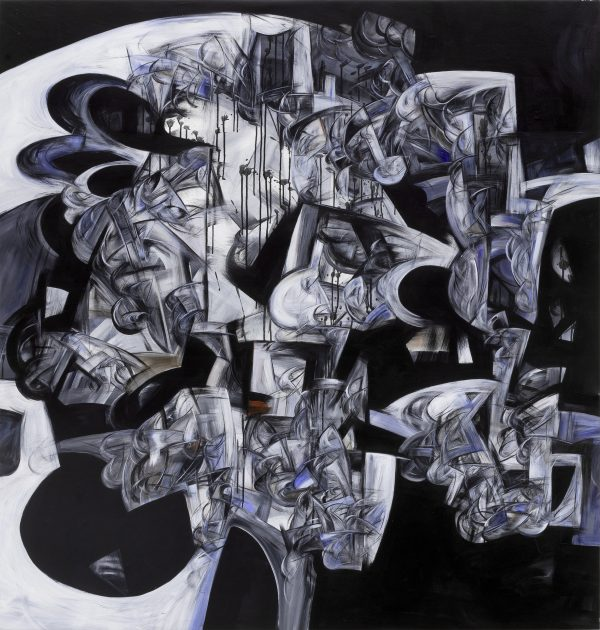2021. 布面丙烯与印度墨. 166×158cm.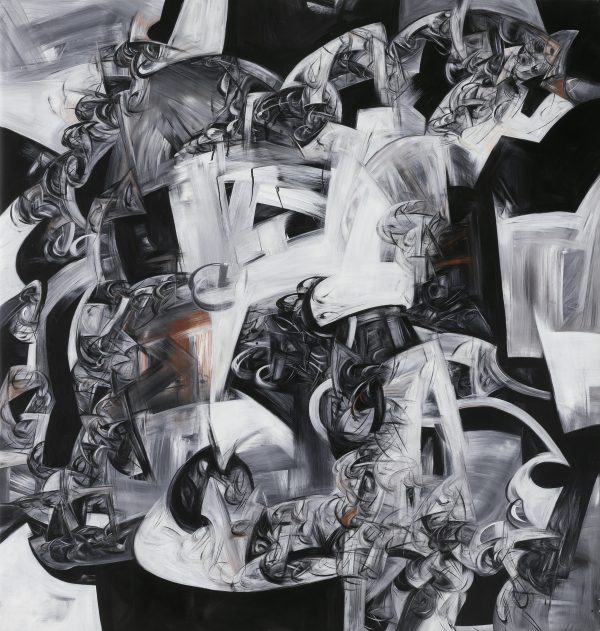2021. 布面丙烯与印度墨. 175×166cm.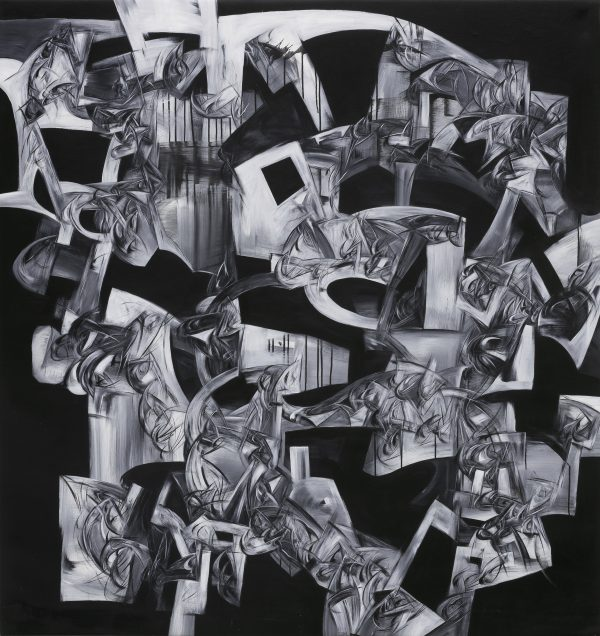2021. 布面丙烯与印度墨. 176×166cm.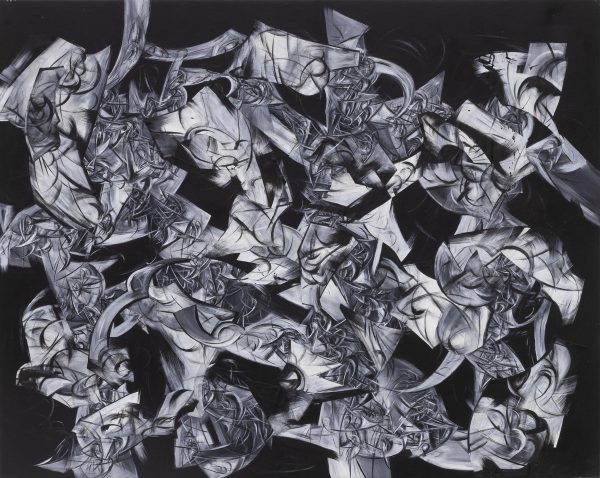2021. 布面丙烯拼贴. 136×171cm.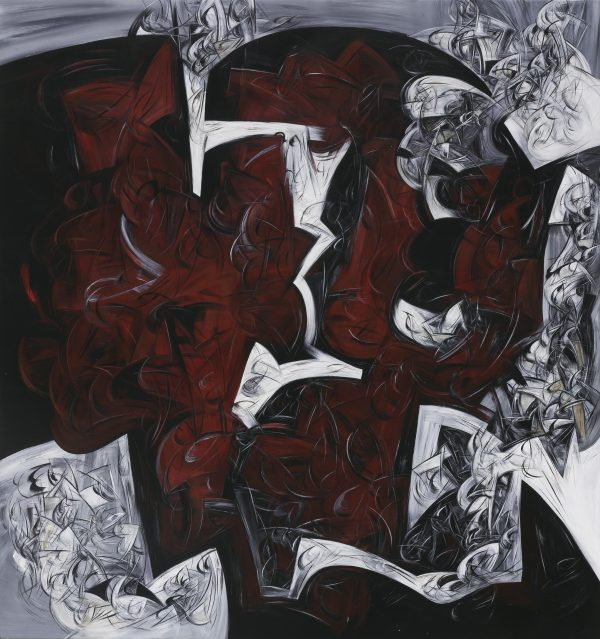2021. 布面丙烯. 157×166cm.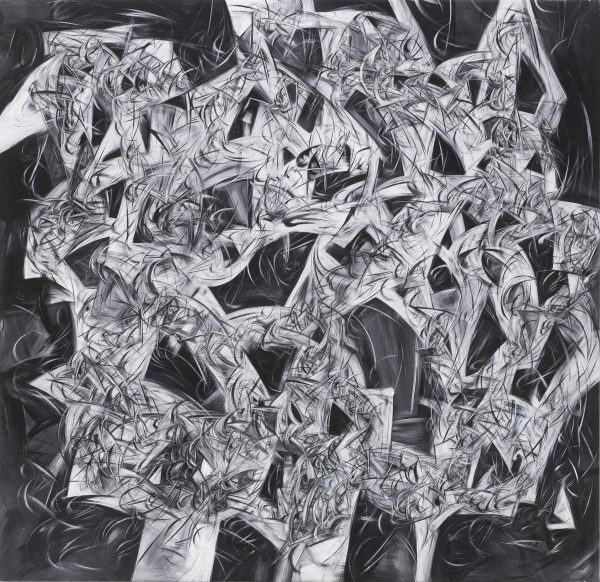2021. 布面丙烯. 162×167cm.2021. 布面丙烯. 167×214cm.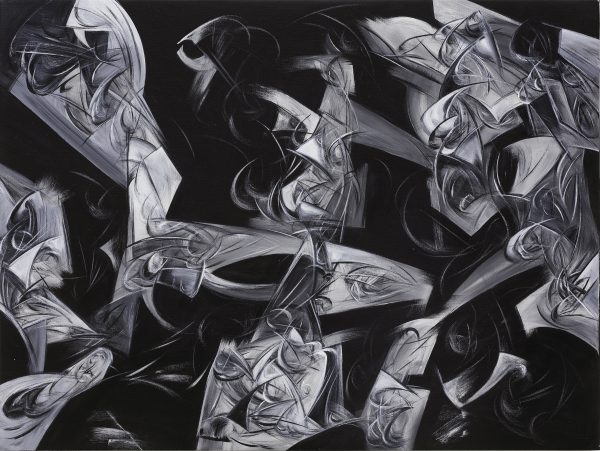Balfron塔

2021. 布面丙烯. 75×100 cm.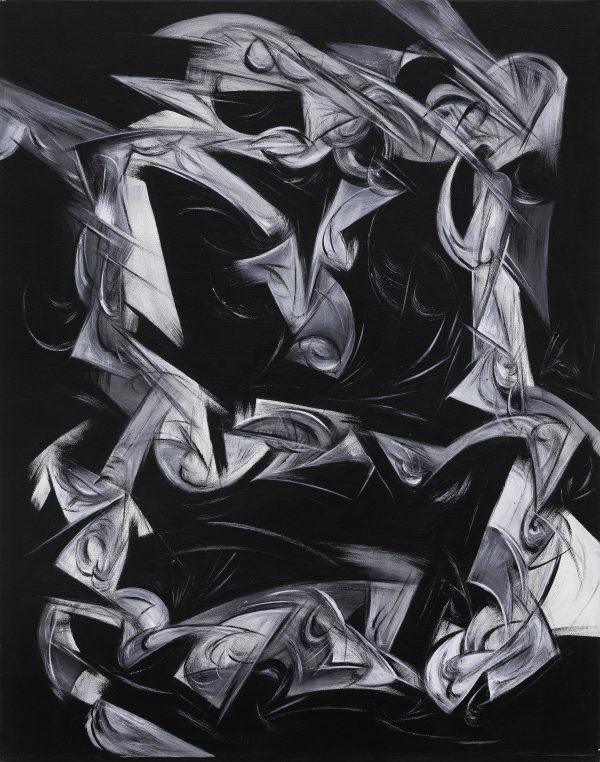2021. 布面丙烯. 76×60cm.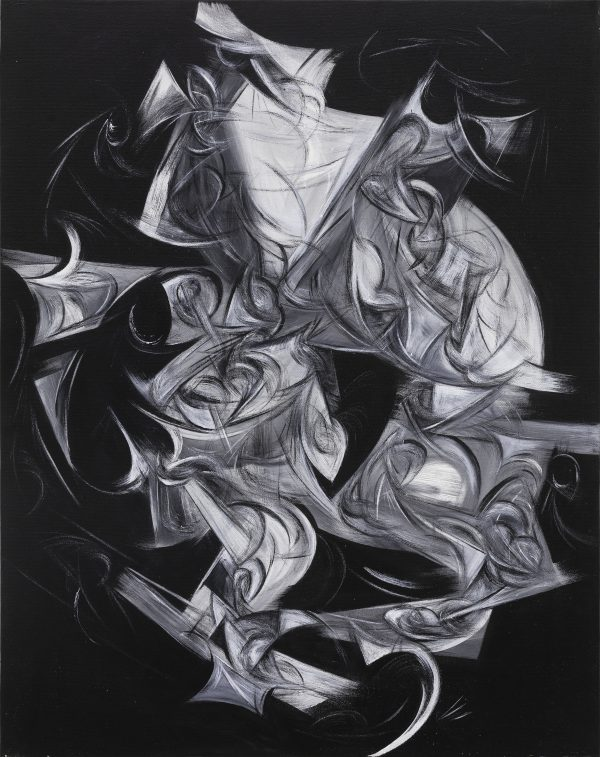2021. 布面丙烯. 76×60cm.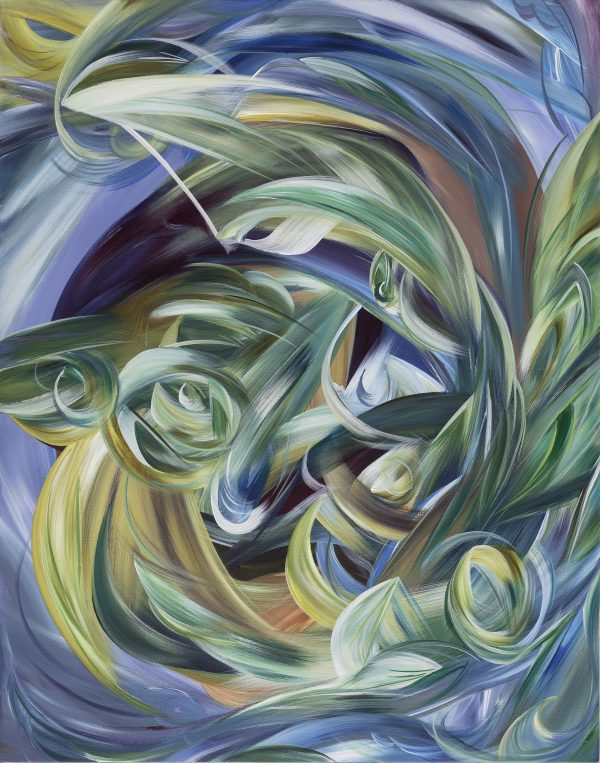2020. 布面丙烯. 70×55cm.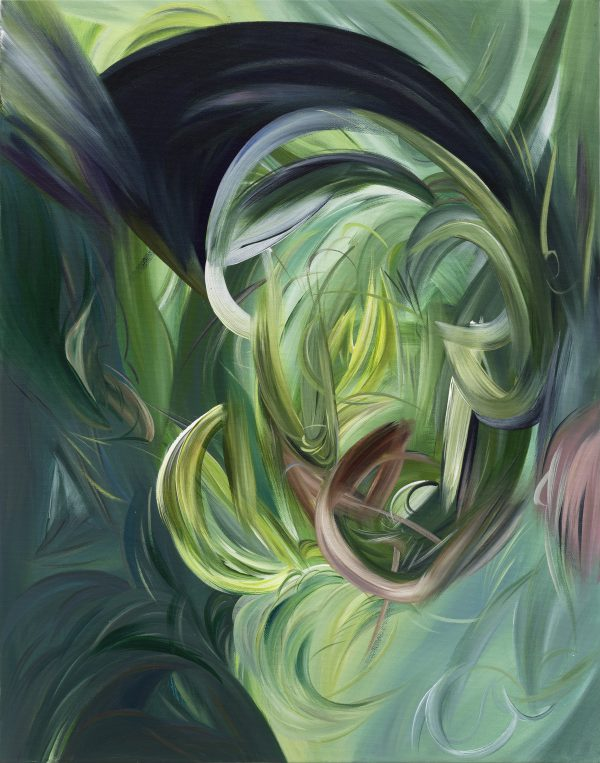2020. 布面丙烯. 70×55cm.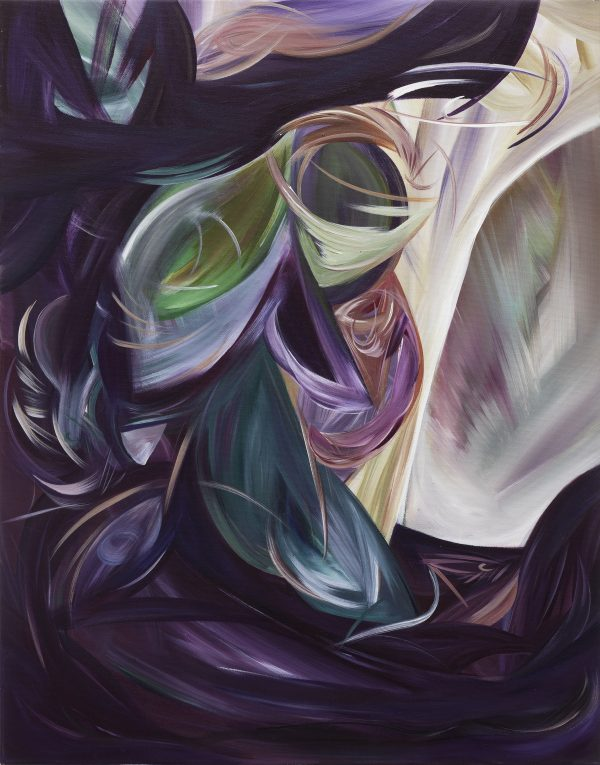2020. 布面丙烯. 70×55cm.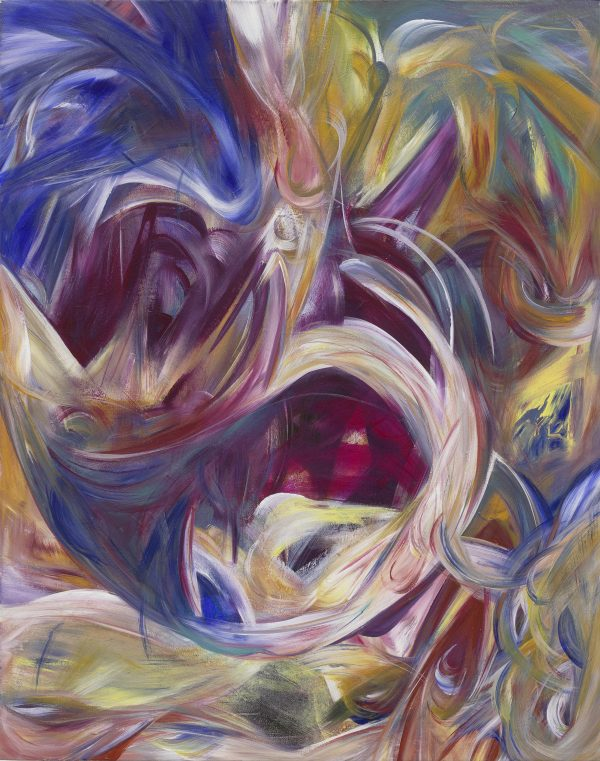2020. 布面丙烯. 75×60cm.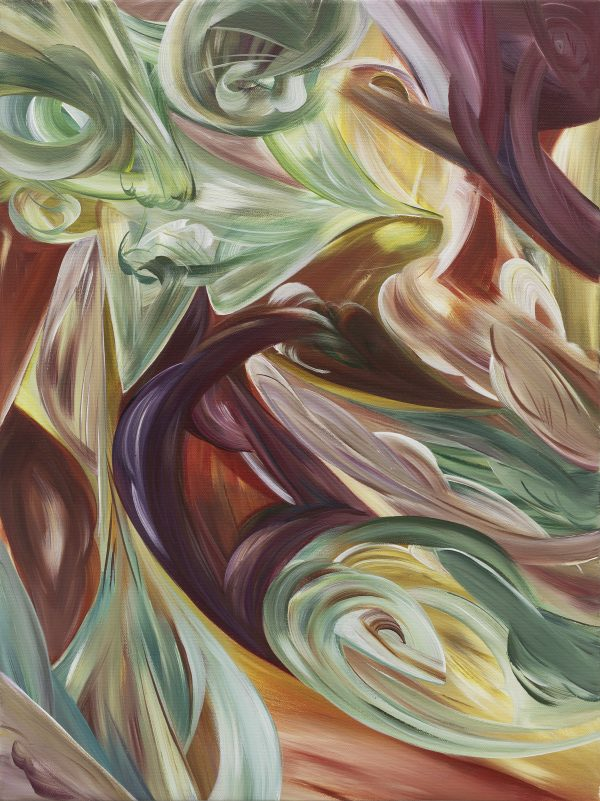2020. 布面丙烯. 60×45cm.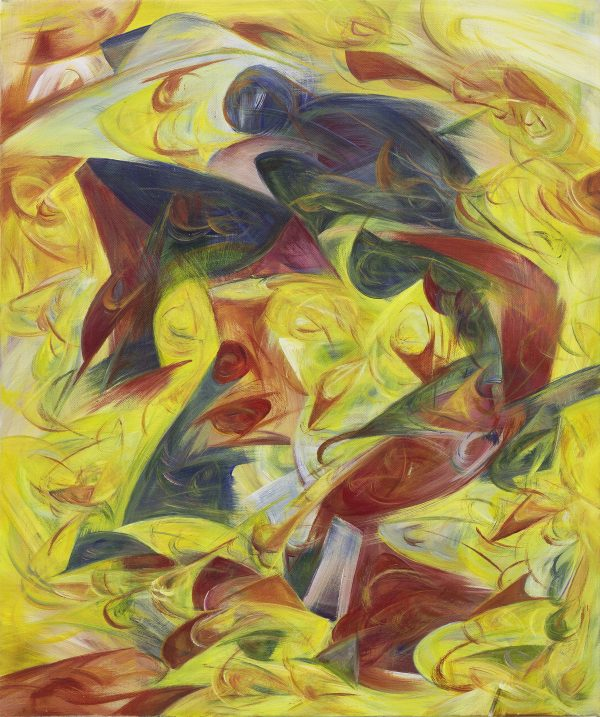2020. 布面丙烯. 60×50cm.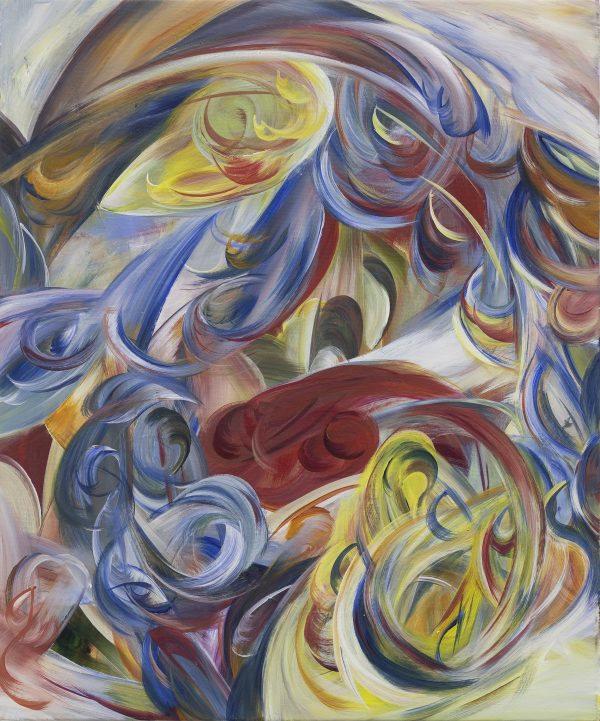2020. 布面丙烯. 60×50cm.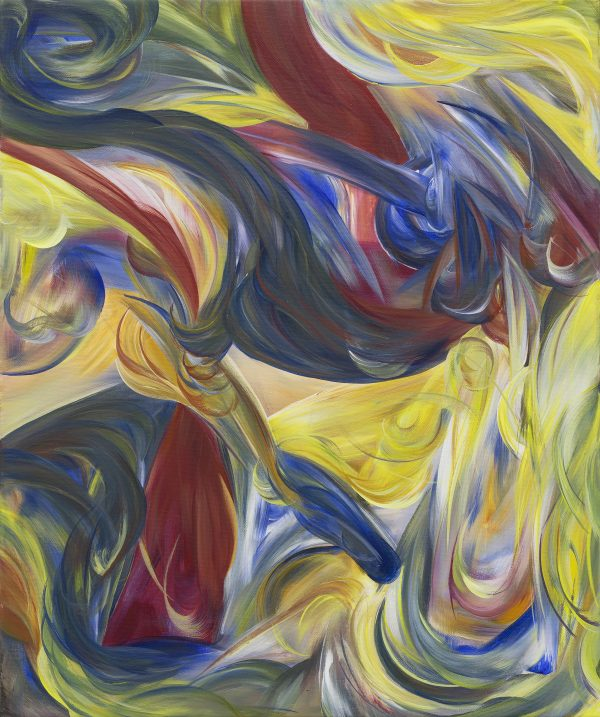2020. 布面丙烯. 60×50cm.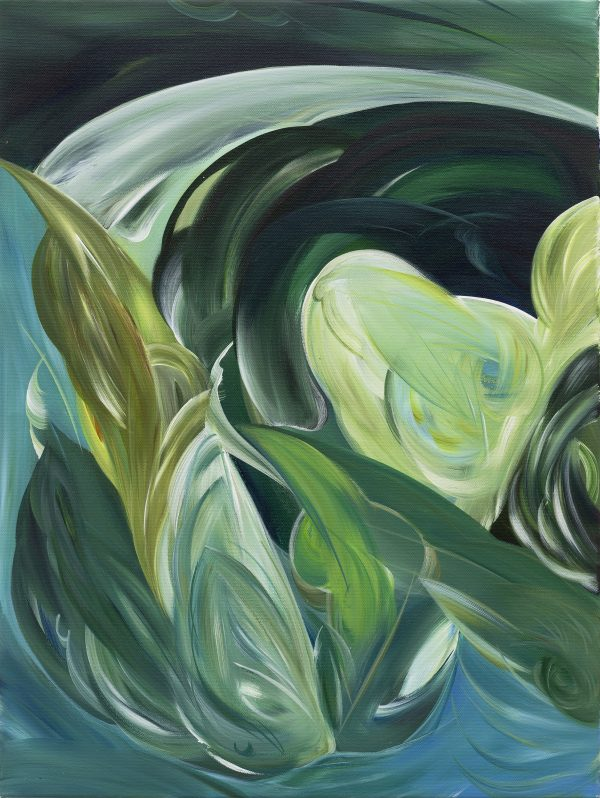2020. 布面丙烯. 60×45cm.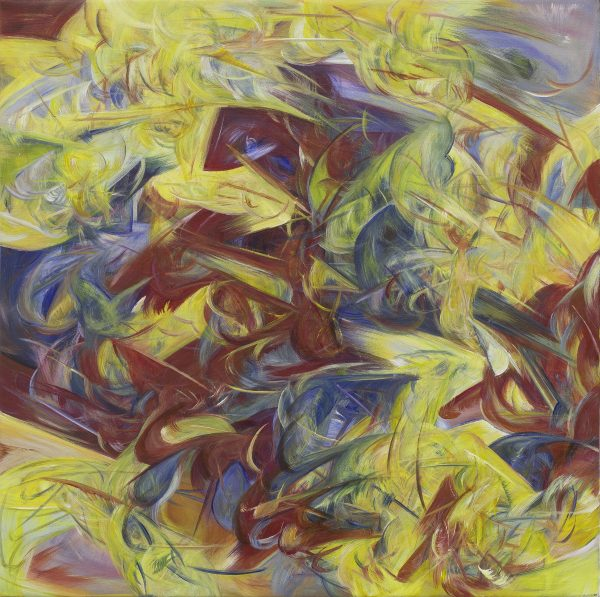2020. 布面丙烯. 60×60cm.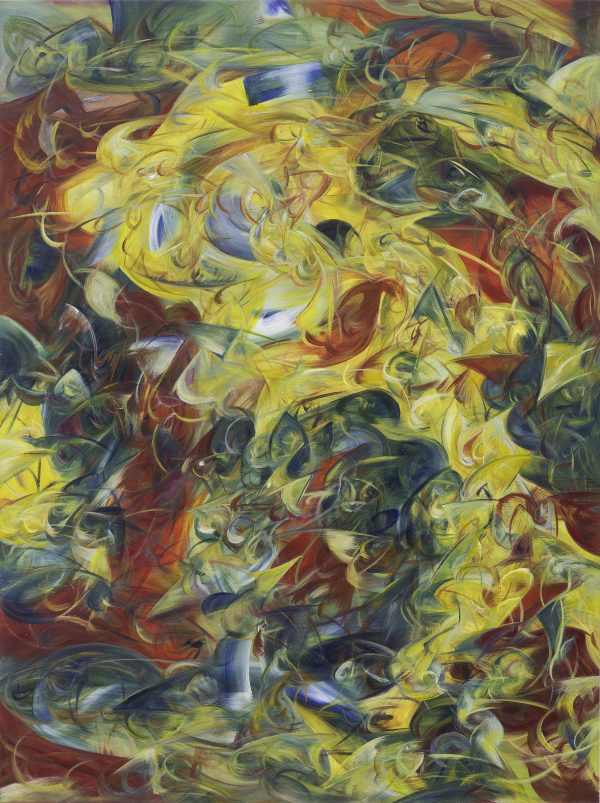2020. 布面丙烯. 100×75cm.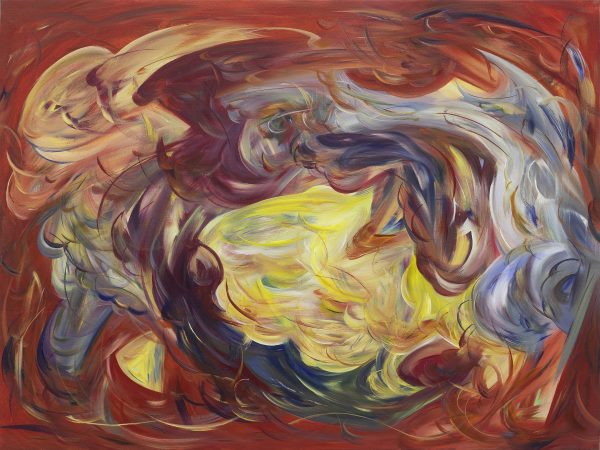2020. 布面丙烯. 75×100cm.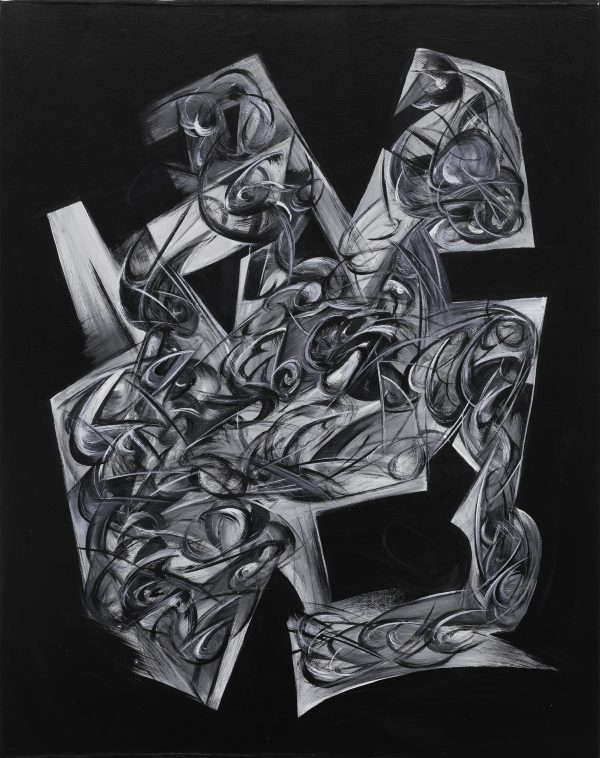2020. 布面丙烯. 76×60cm.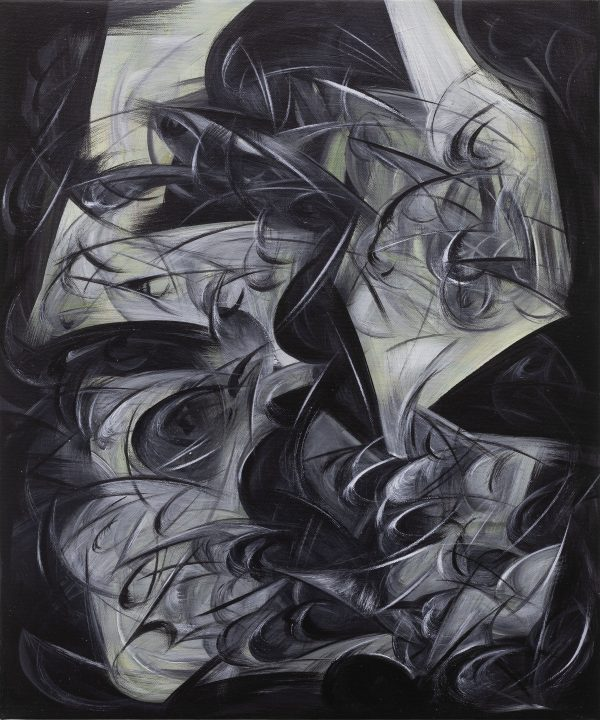2020. 布面丙烯. 60×50cm.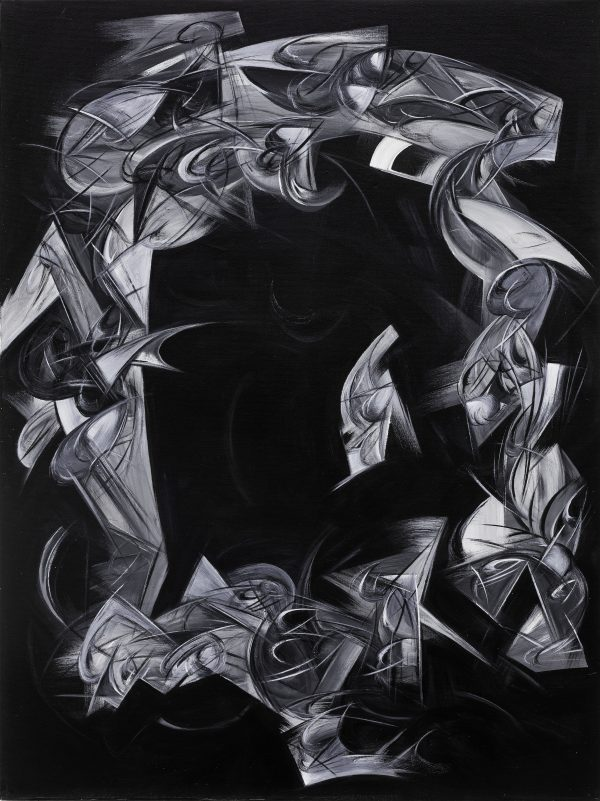2020. 布面丙烯. 100×75cm.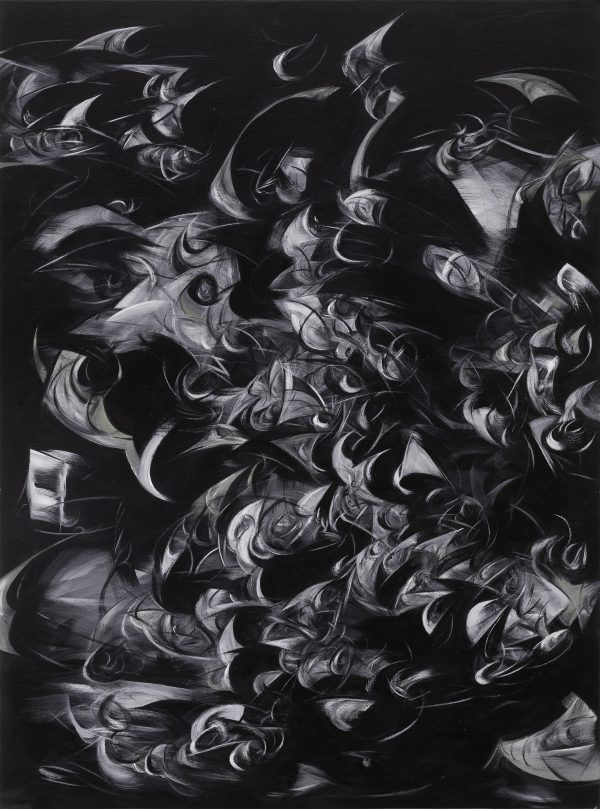2020. 布面丙烯. 121×90cm.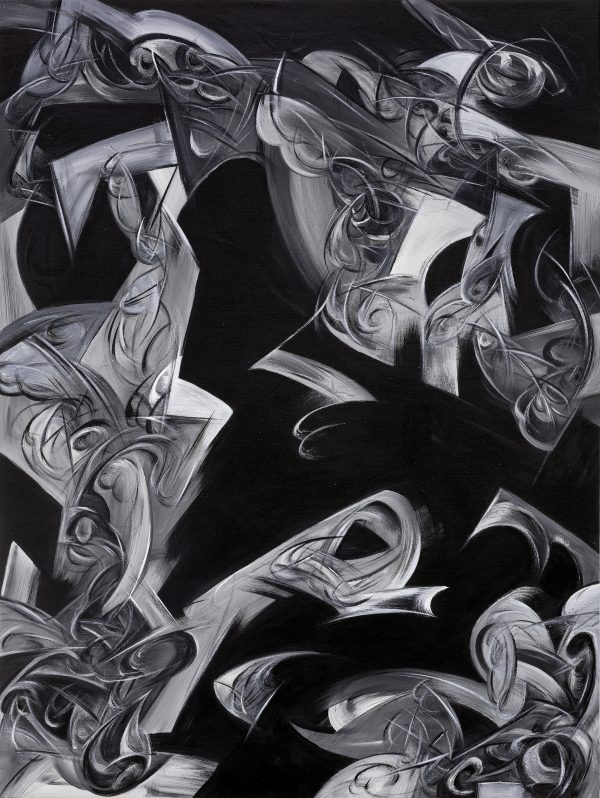2020. 布面丙烯. 100×75cm.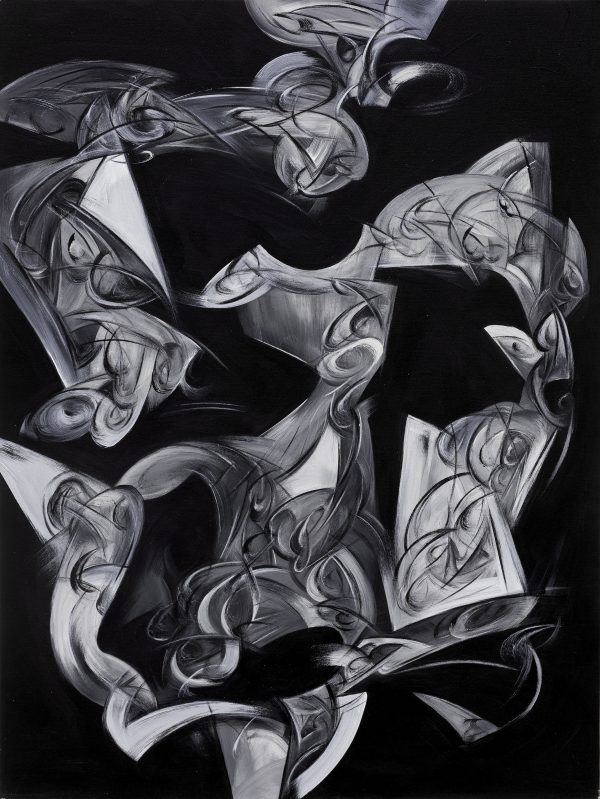2020. 布面丙烯. 100×75cm.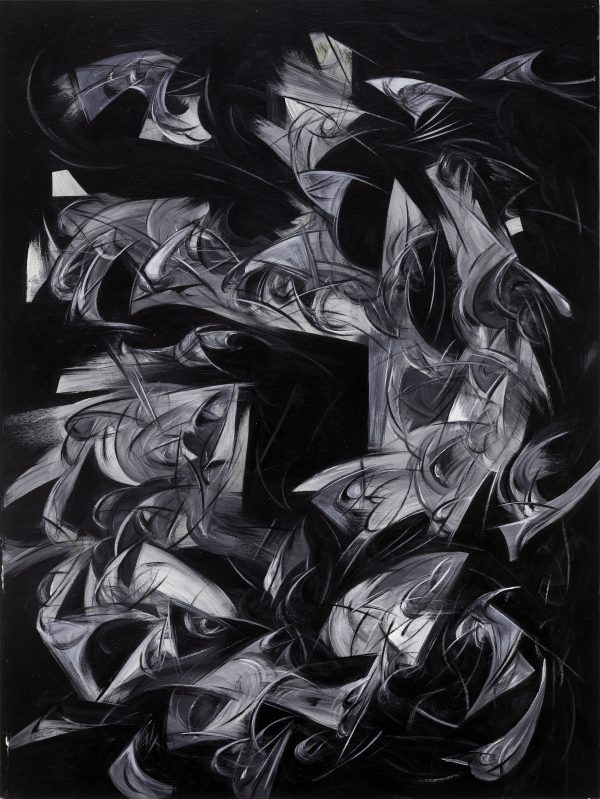2020. 布面丙烯. 100×75cm.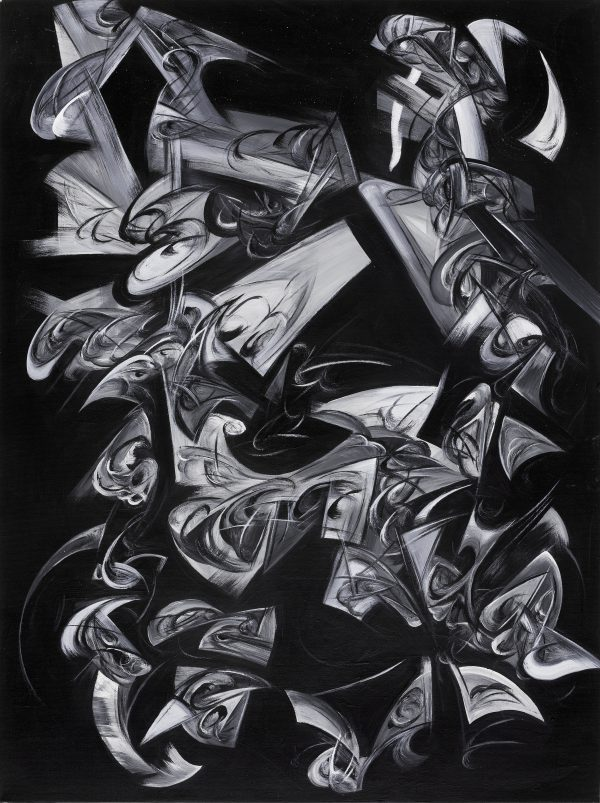2020. 布面丙烯. 100×75 cm.